# scikit-learn学习之SVM算法

======================================================================

======================================================================

# 一：我对SVM的理解

## 1：超平面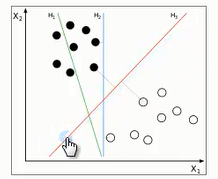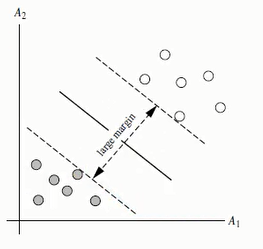## 2：线性可区分（linear separable）和线性不可区分（linear inseparable）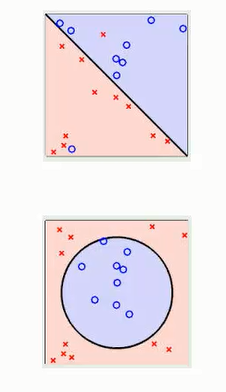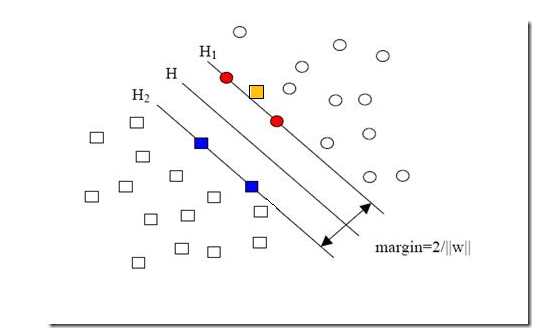## 3：针对线性可区分，求超平面推导H1：H1：w0 + w1 * x1 +w2 * x2 > 1 for yi=+1

H2：w0 + w1 * x1 +w2 * x2  =< -1 for yi=-1

(1): yi ( w0 + w1 * x1 +w2 * x2 ) >= 1  ,对于所有的i来说

W= sqrt（W1^2 + W2^2 + ... + Wn^2）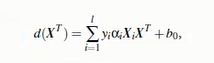yi是支持向量点Xi的类别标记

X^T是要测试的实例

ai和b0都是单一数值型参数

l是支持向量点的个数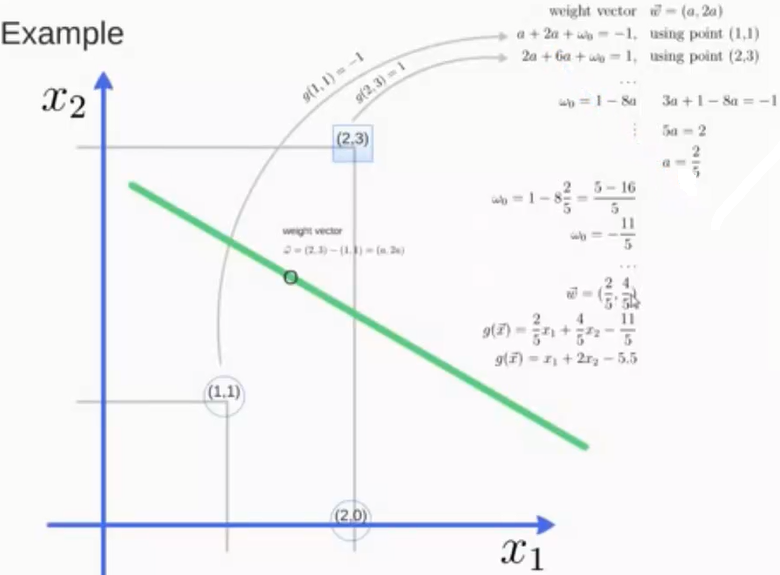SVM训练出的模型完全依赖于支持向量，即使所有训练集里所有非支持向量的点都被去除，重复训练过程，结果仍会得到一个完全一模一样的模型

一个SVM如果训练得出的支持向量个数比较小，SVM训练出的模型也容易被泛化

## 4：针对线性不可区分，求超平面推导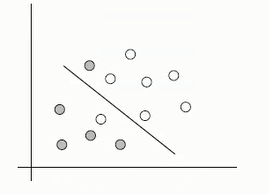1：利用一个非线性的映射把原数据集中的向量点转化到一个更高维的空间中

2：在这个高纬度的空间中找一个线性超平面来根据线性可分的情况处理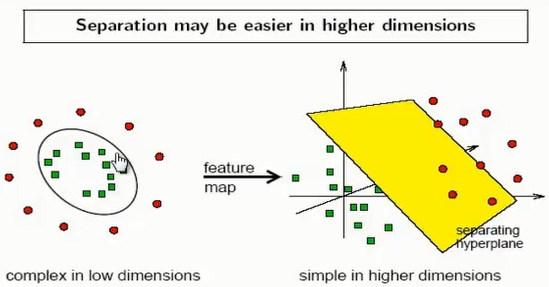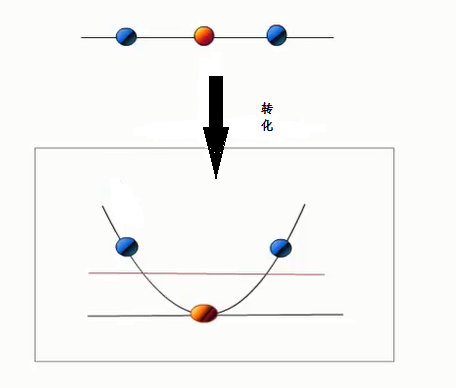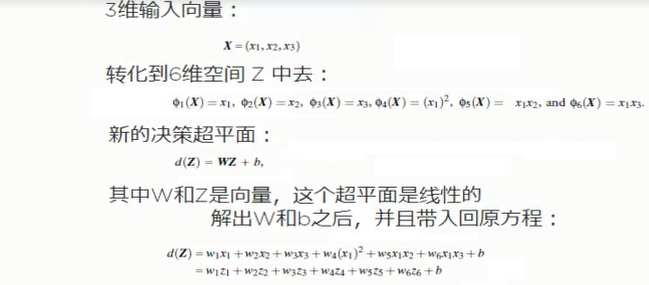## 5：核函数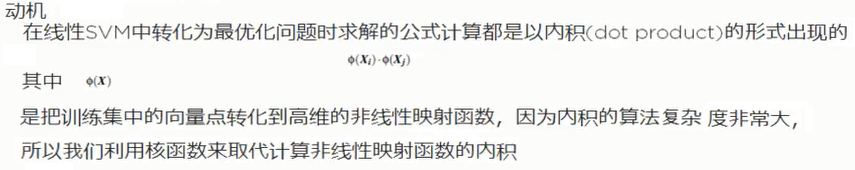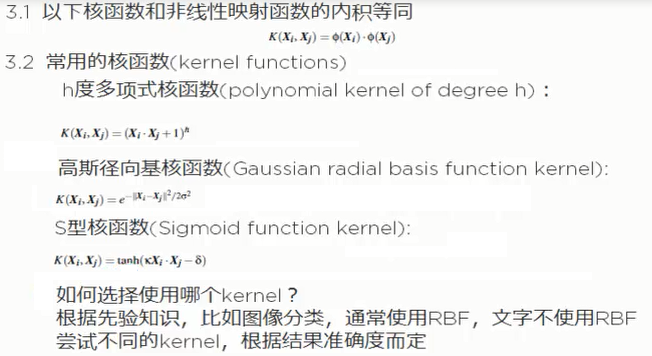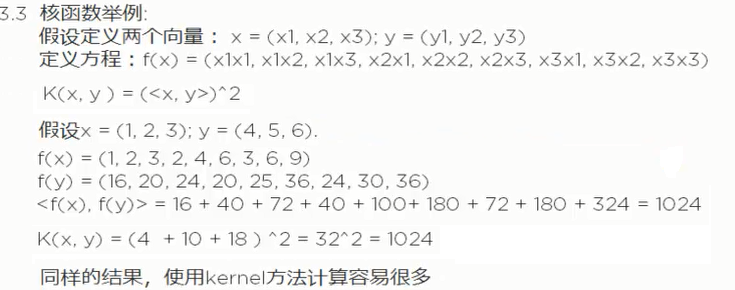# 二：Scikit-learn上对SVM相关描述

## 1：Classification

### 首先我们来看看SVC，NvSVC，LinearSVC的区别和样例

SVC（C-Support Vector Classification）：支持向量分类，基于libsvm实现的（libsvm详情参考 或者百科），数据拟合的时间复杂度是数据样本的二次方，这使得他很难扩展到10000个数据集，当输入是多类别时（SVM最初是处理二分类问题的），通过一对一的方案解决，当然也有别的解决办法，比如说（以下为引用）：

========================================================================================

SVM解决多分类问题的方法
SVM算法最初是为二值分类问题设计的，当处理多类问题时，就需要构造合适的多类分类器。目前，构造SVM多类分类器的方法主要有两类：一类是直接法，直接在目标函数上进行修改，将多个分类面的参数求解合并到一个最优化问题中，通过求解该最优化问题“一次性”实现多类分类。这种方法看似简单，但其计算复杂度比较高，实现起来比较困难，只适合用于小型问题中；另一类是间接法，主要是通过组合多个二分类器来实现多分类器的构造，常见的方法有one-against-one和one-against-all两种。
a.一对多法（one-versus-rest,简称1-v-r SVMs）。训练时依次把某个类别的样本归为一类,其他剩余的样本归为另一类，这样k个类别的样本就构造出了k个SVM。分类时将未知样本分类为具有最大分类函数值的那类。
b.一对一法（one-versus-one,简称1-v-1 SVMs）。其做法是在任意两类样本之间设计一个SVM，因此k个类别的样本就需要设计k(k-1)/2个SVM。当对一个未知样本进行分类时，最后得票最多的类别即为该未知样本的类别。Libsvm中的多类分类就是根据这个方法实现的。
c.层次支持向量机（H-SVMs）。层次分类法首先将所有类别分成两个子类，再将子类进一步划分成两个次级子类，如此循环，直到得到一个单独的类别为止。

d.其他多类分类方法。除了以上几种方法外，还有有向无环图SVM（Directed Acyclic Graph SVMs，简称DAG-SVMs）和对类别进行二进制编码的纠错编码SVMs。

=====================================================================================
svc使用代码示例（我演示的是最简单的，官网上还有很多看起来很漂亮的分类示例，感兴趣的可以自己参考下）：



<span style=
"font-family:Microsoft YaHei;"><span style=
"font-family:Microsoft YaHei;font-size:14px;">
'''

SVC参数解释

（1）C: 目标函数的惩罚系数C，用来平衡分类间隔margin和错分样本的，default C = 1.0；

（2）kernel：参数选择有RBF, Linear, Poly, Sigmoid, 默认的是"RBF";

（3）degree：if you choose 'Poly' in param 2, this is effective, degree决定了多项式的最高次幂；

（4）gamma：核函数的系数('Poly', 'RBF' and 'Sigmoid'), 默认是gamma = 1 / n_features;

（5）coef0：核函数中的独立项，'RBF' and 'Poly'有效；

（6）probablity: 可能性估计是否使用(true or false)；

（7）shrinking：是否进行启发式；

（8）tol（default = 1e - 3）: svm结束标准的精度;

（9）cache_size: 制定训练所需要的内存（以MB为单位）；

（10）class_weight: 每个类所占据的权重，不同的类设置不同的惩罚参数C, 缺省的话自适应；

（11）verbose: 跟多线程有关，不大明白啥意思具体；

（12）max_iter: 最大迭代次数，default = 1， if max_iter = -1, no limited;

（13）decision_function_shape ： ‘ovo’ 一对一, ‘ovr’ 多对多  or None 无, default=None

（14）random_state ：用于概率估计的数据重排时的伪随机数生成器的种子。

ps：7,8,9一般不考虑。

'''

from sklearn.svm
import SVC

import numpy
as np

X= np.array([[
-1,
-1],[
-2,
-1],[
1,
1],[
2,
1]])

y = np.array([
1,
1,
2,
2])

clf = SVC()

clf.fit(X,y)

print clf.fit(X,y)

print clf.predict([[
-0.8,
-1]])</span></span>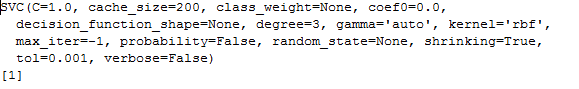NuSVC（Nu-Support Vector Classification.）：核支持向量分类，和SVC类似，也是基于libsvm实现的，但不同的是通过一个参数空值支持向量的个数



<span style=
"font-family:Microsoft YaHei;"><span style=
"font-family:Microsoft YaHei;font-size:14px;">
'''

NuSVC参数

nu：训练误差的一个上界和支持向量的分数的下界。应在间隔（0，1 ]。

其余同SVC

'''

import numpy
as np

X = np.array([[
-1,
-1], [
-2,
-1], [
1,
1], [
2,
1]])

y = np.array([
1,
1,
2,
2])

from sklearn.svm
import NuSVC

clf = NuSVC()

clf.fit(X, y)

print clf.fit(X,y)

print(clf.predict([[
-0.8,
-1]]))

</span></span>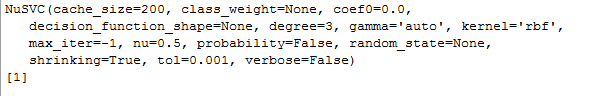LinearSVC（Linear Support Vector Classification）：线性支持向量分类，类似于SVC，但是其使用的核函数是”linear“上边介绍的两种是按照brf（径向基函数计算的，其实现也不是基于LIBSVM，所以它具有更大的灵活性在选择处罚和损失函数时，而且可以适应更大的数据集，他支持密集和稀疏的输入是通过一对一的方式解决的



<span style=
"font-family:Microsoft YaHei;">
'''

LinearSVC 参数解释

C：目标函数的惩罚系数C，用来平衡分类间隔margin和错分样本的，default C = 1.0；

loss ：指定损失函数

penalty ：

dual ：选择算法来解决对偶或原始优化问题。当n_samples > n_features 时dual=false。

tol ：（default = 1e - 3）: svm结束标准的精度;

multi_class：如果y输出类别包含多类，用来确定多类策略， ovr表示一对多，“crammer_singer”优化所有类别的一个共同的目标

如果选择“crammer_singer”，损失、惩罚和优化将会被被忽略。

fit_intercept ：

intercept_scaling ：

class_weight ：对于每一个类别i设置惩罚系数C = class_weight[i]*C,如果不给出，权重自动调整为 n_samples / (n_classes * np.bincount(y))

verbose：跟多线程有关，不大明白啥意思具体<pre name="code" class="python">

from sklearn.svm import SVC

X=[,,,]

Y = [0,1,2,3]

clf = SVC(decision_function_shape='ovo') #ovo为一对一

clf.fit(X,Y)

print clf.fit(X,Y)

dec = clf.decision_function([])    #返回的是样本距离超平面的距离

print dec

clf.decision_function_shape = "ovr"

dec =clf.decision_function() #返回的是样本距离超平面的距离

print dec

#预测

print clf.predict()</span>



random_state ：用于概率估计的数据重排时的伪随机数生成器的种子。max_iter ：'''import numpy as npX = np.array([[-1, -1], [-2, -1], [1, 1], [2, 1]])y = np.array([1, 1, 2, 2])from sklearn.svm import LinearSVCclf = LinearSVC()clf.fit(X, y) print clf.fit(X,y)print(clf.predict([[-0.8, -1]]))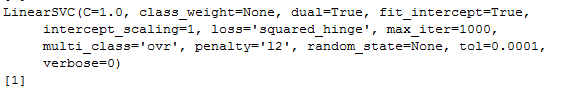### Multi-class classification（多类别分类）

SVC和NuSVC对于多分类问题采用的是一对一的方法，若n_class表示类别的数目，则需要构造n_class *（n_class - 1）/ 2个分类器，每一次训练集采用两类别，提供了一个与其它分类一致的接口，该decision_function_shape选项允许聚合结果的“一对一”的分类决策函数的形状，下边看一小例子



<span style=
"font-family:Microsoft YaHei;">
#-*-coding:utf-8-*-

'''

Created on 2016年4月29日

@author: Gamer Think

'''

from sklearn.svm
import SVC,LinearSVC

X=[[
0],[
1],[
2],[
3]]

Y = [
0,
1,
2,
3]

'''

SVC and NuSVC

'''

clf = SVC(decision_function_shape=
'ovo')
#ovo为一对一

clf.fit(X,Y)

print
"SVC:",clf.fit(X,Y)

dec = clf.decision_function([[
1]])
#返回的是样本距离超平面的距离

print
"SVC:",dec

clf.decision_function_shape =
"ovr"

dec =clf.decision_function([
1])
#返回的是样本距离超平面的距离

print
"SVC:",dec

#预测

print
"预测：",clf.predict([
1])

'''</span><pre name="code" class="python"><span style="font-family:Microsoft YaHei;">LinearSVC</span>


'''lin_clf = LinearSVC()lin_clf.fit(X, Y) dec = lin_clf.decision_function([])print "LinearSVC:",dec.shape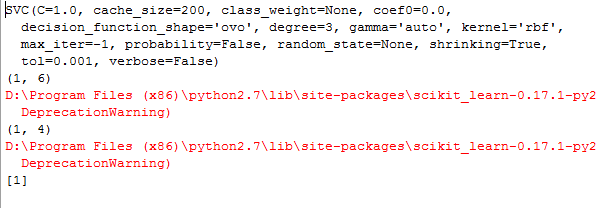### Unbalanced problems（数据不平衡问题）



<span style=
"font-family:Microsoft YaHei;">
# -*-coding:utf-8-*-

'''

Created on 2016年5月4日

@author: Gamer Think

'''

import numpy
as np

import matplotlib.pyplot
as plt

from sklearn
import svm

#from sklearn.linear_model import SGDClassifier

# we create 40 separable points

rng = np.random.RandomState(
0)

n_samples_1 =
1000

n_samples_2 =
100

X = np.r_[
1.5 * rng.randn(n_samples_1,
2),
0.5 * rng.randn(n_samples_2,
2) + [
2,
2]]

y = [
0] * (n_samples_1) + [
1] * (n_samples_2)

print X

print y

# fit the model and get the separating hyperplane

clf = svm.SVC(kernel=
'linear', C=
1.0)

clf.fit(X, y)

w = clf.coef_[
0]

a = -w[
0] / w[
1]
#a可以理解为斜率

xx = np.linspace(
-5,
5)

yy = a * xx - clf.intercept_[
0] / w[
1]
#二维坐标下的直线方程

# get the separating hyperplane using weighted classes

wclf = svm.SVC(kernel=
'linear', class_weight={
1:
10})

wclf.fit(X, y)

ww = wclf.coef_[
0]

wa = -ww[
0] / ww[
1]

wyy = wa * xx - wclf.intercept_[
0] / ww[
1]
#带权重的直线

# plot separating hyperplanes and samples

h0 = plt.plot(xx, yy,
'k-', label=
'no weights')

h1 = plt.plot(xx, wyy,
'k--', label=
'with weights')

plt.scatter(X[:,
0], X[:,
1], c=y)

plt.legend()

plt.axis(
'tight')

plt.show()</span>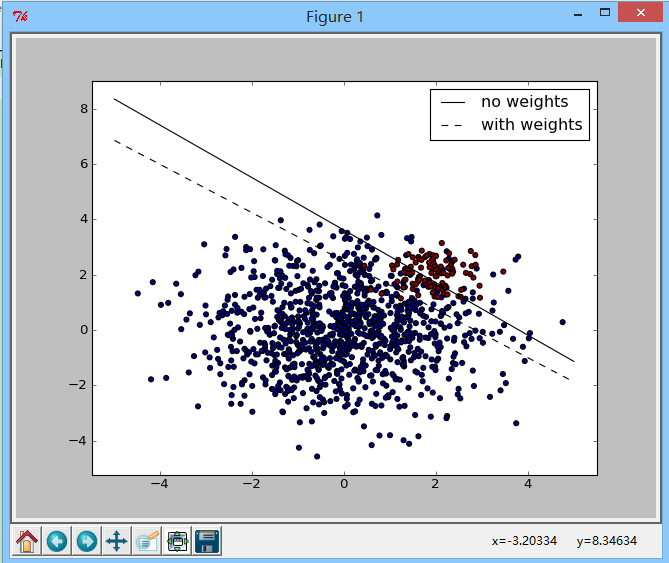## 2：Regression



<span style=
"font-family:Microsoft YaHei;">>>>
from sklearn
import svm

>>> X = [[
0,
0], [
2,
2]]

>>> y = [
0.5,
2.5]

>>> clf = svm.SVR()

>>> clf.fit(X, y)

SVR(C=
1.0, cache_size=
200, coef0=
0.0, degree=
3, epsilon=
0.1, gamma=
'auto',

kernel=
'rbf', max_iter=
-1, shrinking=
True, tol=
0.001, verbose=
False)

>>> clf.predict([[
1,
1]])

array([
1.5])</span>





<span style=
"font-family:Microsoft YaHei;">
#-*-coding:utf-8-*-

'''

Created on 2016年5月4日

@author: Gamer Think

'''

import numpy
as np

from sklearn.svm
import SVR

import matplotlib.pyplot
as plt

###############################################################################

# Generate sample data

X = np.sort(
5 * np.random.rand(
40,
1), axis=
0)
#产生40组数据，每组一个数据，axis=0决定按列排列，=1表示行排列

y = np.sin(X).ravel()
#np.sin()输出的是列，和X对应，ravel表示转换成行

###############################################################################

y[::
5] +=
3 * (
0.5 - np.random.rand(
8))

###############################################################################

# Fit regression model

svr_rbf = SVR(kernel=
'rbf', C=
1e3, gamma=
0.1)

svr_lin = SVR(kernel=
'linear', C=
1e3)

svr_poly = SVR(kernel=
'poly', C=
1e3, degree=
2)

y_rbf = svr_rbf.fit(X, y).predict(X)

y_lin = svr_lin.fit(X, y).predict(X)

y_poly = svr_poly.fit(X, y).predict(X)

###############################################################################

# look at the results

lw =
2

plt.scatter(X, y, color=
'darkorange', label=
'data')

plt.hold(
'on')

plt.plot(X, y_rbf, color=
'navy', lw=lw, label=
'RBF model')

plt.plot(X, y_lin, color=
'c', lw=lw, label=
'Linear model')

plt.plot(X, y_poly, color=
'cornflowerblue', lw=lw, label=
'Polynomial model')

plt.xlabel(
'data')

plt.ylabel(
'target')

plt.title(
'Support Vector Regression')

plt.legend()

plt.show()</span>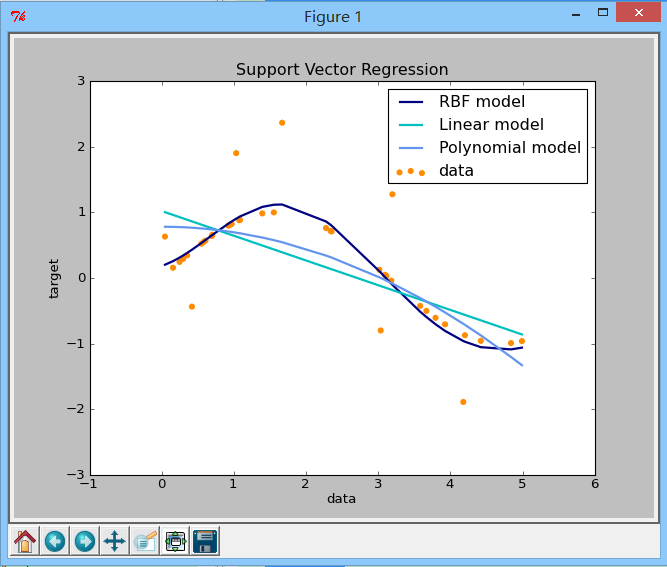SVM实在是很强大，不是我这等菜鸟短时间内所能参透的，以后有机会还会继续学习SVM，我相信不同的时间看同一个算法或者项目会有不同的收获，谢谢
10-23809
12-19574
04-241841
03-212429
10-07934
09-15441
09-16508
08-06147
09-16206
08-06227
09-045146
01-30966
02-15214
05-221880
09-01202
04-043618
03-072361
07-23807
11-261525

### “相关推荐”对你有帮助么？

•非常没帮助
•没帮助
•一般
•有帮助
•非常有帮助被折叠的  条评论 为什么被折叠?到【灌水乐园】发言点击重新获取扫码支付1.余额是钱包充值的虚拟货币，按照1:1的比例进行支付金额的抵扣。
2.余额无法直接购买下载，可以购买VIP、C币套餐、付费专栏及课程。余额充值